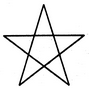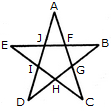# Non Verbal Reasoning - Analytical Reasoning - Discussion

Discussion Forum : Analytical Reasoning - Section 1 (Q.No. 13)
13.

Find the number of triangles in the given figure.5
6
8
10
Explanation:

The figure may be labelled as shown.The simplest triangles are AJF, FBG, GCH, HDI and IEJ i.e. 5 in number.

The triangles composed of three components each EBH, AIC, EFC, ADG and BJD i.e. 5 in number.

Thus, there are 5 + 5 = 10 triangles in the figure.

Discussion:
8 comments Page 1 of 1.

Mithila said:   5 years ago
The answer is 8. There are no triangles composed of three components.

There are 5 simple triangles and 3 triangles composed of two components.

Gopal said:   4 years ago

Mythili said:   3 years ago

Because each triangle has three components with contains of A, B, C. So there is an 10 number of triangles.

Prashanthy said:   3 years ago
According to me, the answer is 6.

Prasanna said:   3 years ago